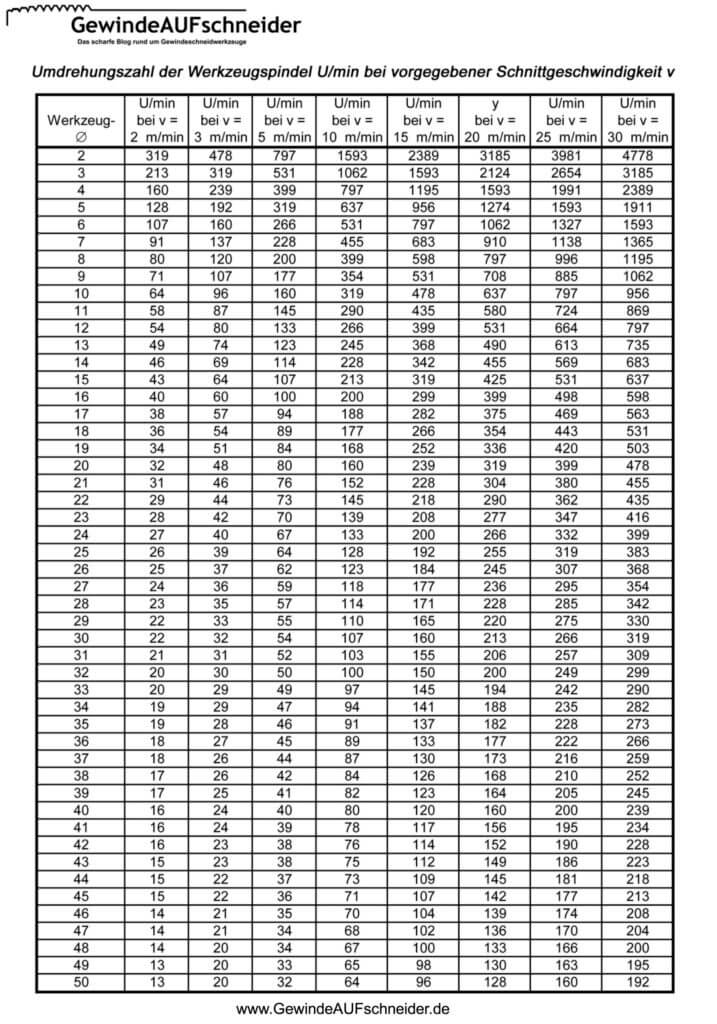• ## Conversion table:

###The table is based on the formula v = d x Pi x n /1000, where d must be entered in mm.
If you change the formula, n = v x 1000/dxPi. The cutting speed v should be 25 m/min.
The diameter of the tool M 6 is 6 mm. This gives 25 x 1000 = 25000 ; 6 x 3.1415 = 18.84 ; 25000 / 18.84 = 1326.96 .
These are the necessary revolutions of the machine at the cutting speed of 25 m/min